Next: Logic Gates Up: Digital Circuits Previous: Number Representation

# Boolean Algebra

The binary 0 and 1 states are naturally related to the true and false logic variables. We will find the following Boolean algebra useful. Consider two logic variables A and B and the result of some Boolean logic operation Q. We can define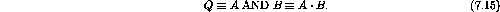Q is true if and only if A is true AND B is true.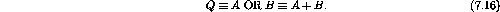Q is true if A is true OR B is true.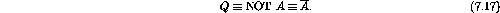Q is true if A is false.

A useful way of displaying the results of a Boolean operation is with a truth table. We will make extensive use of truth tables later. If no ``-'' is available on your text processor or circuit drawing program an ``N'' can be used, ie.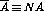.

We list a few trivial Boolean rules in table 7.2.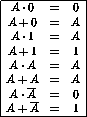Table 7.2:  Properties of Boolean Operations.

The Boolean operations obey the usual commutative, distributive and associative rules of normal algebra (table 7.3).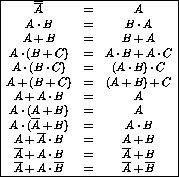Table 7.3:  Boolean commutative, distributive and associative rules.

We will also make extensive use of De Morgan's theorems (table 7.4).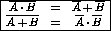Table 7.4:  De Morgan's theorems.

Doug Gingrich
Tue Jul 13 16:55:15 EDT 1999